# Use These Easy Homemade Spinners to Practice Fractions

Mastering fractions one spin at a time.

Many elementary teachers have told us that fractions are one of the hardest math skills for kids to master. That’s why we created these free fraction games, to go along with our free multiplication games. Our packet of free fractions worksheets includes 11 printable games. Students can play the games to practice writing and recognizing fractions, coloring and producing fractions, fraction equivalents, and fraction-decimal conversion.

## Here’s how it works

### 1. Save and print our free fractions worksheets printable packet.

It includes 11 free fractions worksheets for different levels, from beginners to advanced. Plus: answer keys!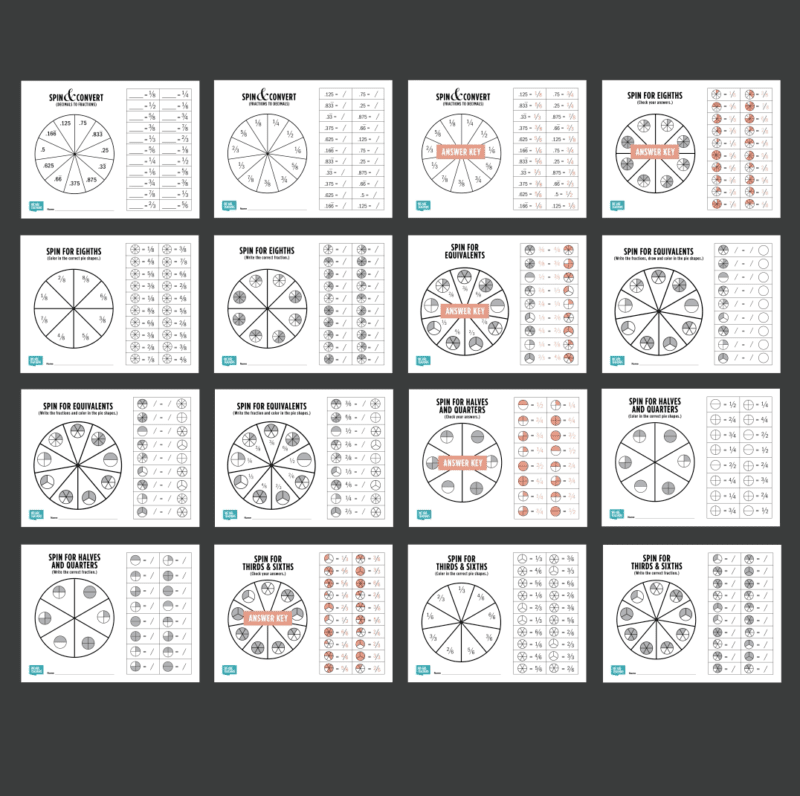### 2. Choose the fraction set or skill that you want students to practice and distribute that sheet.

The packet includes games to help recognize and write fractions, produce and color fractions, practice fraction equivalents, and work on fraction-decimal conversion.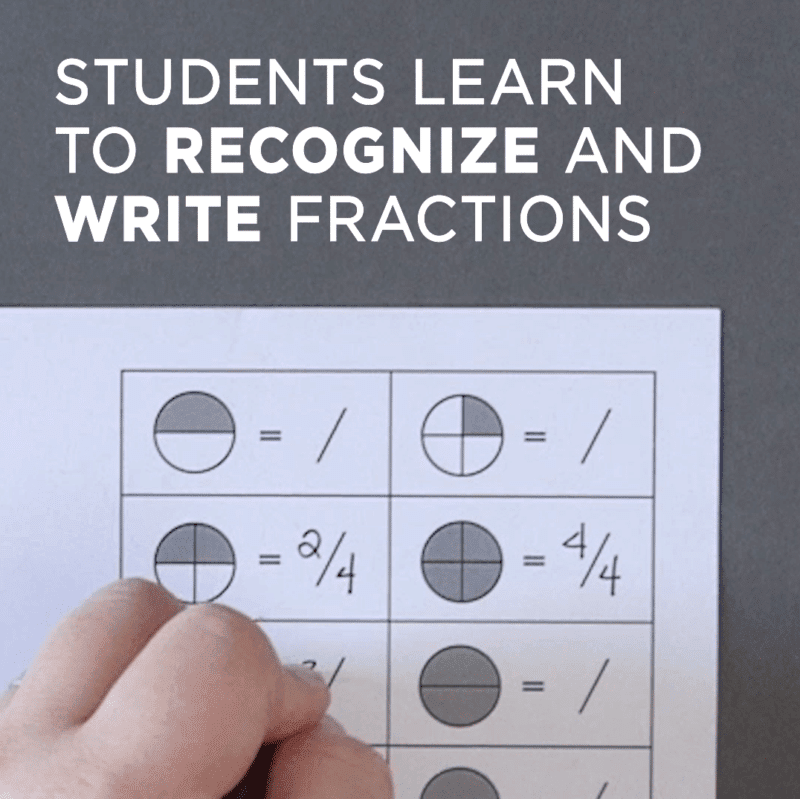### 3. Give each student a pencil and a paper clip to create a simple spinner.

Have students spin for a fraction to write, reproduce, or convert, depending on the worksheet.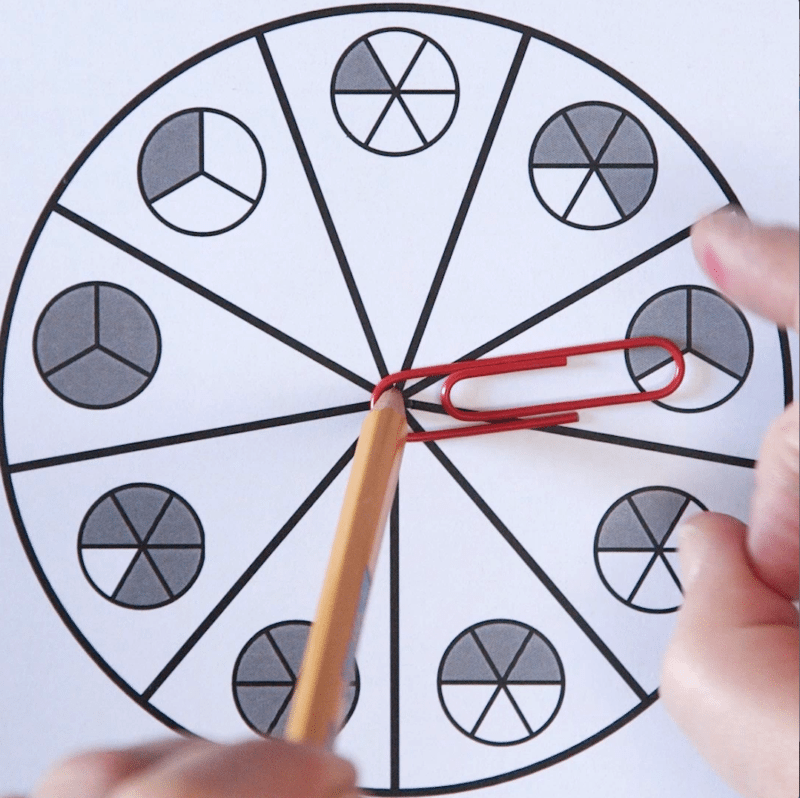### 4. As students become more confident with their fractions skills, give them the more challenging fractions worksheets.

This is the fractions to decimals conversion game.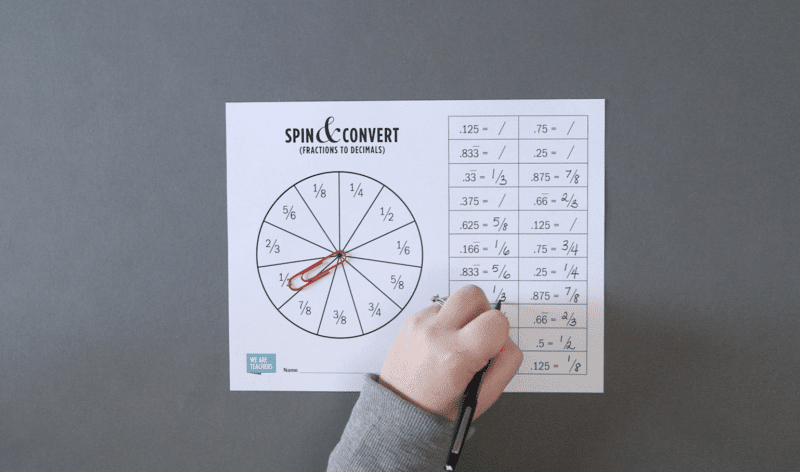## Ready to try it yourself? Get our free fractions worksheets printable packet now!

Number lines are terrific for estimation, too. Find 18 estimation activities for your classroom here.

10 frames are another incredibly useful math tool. Here are some fantastic and fun 10 Frame Activities you’ll want to try next.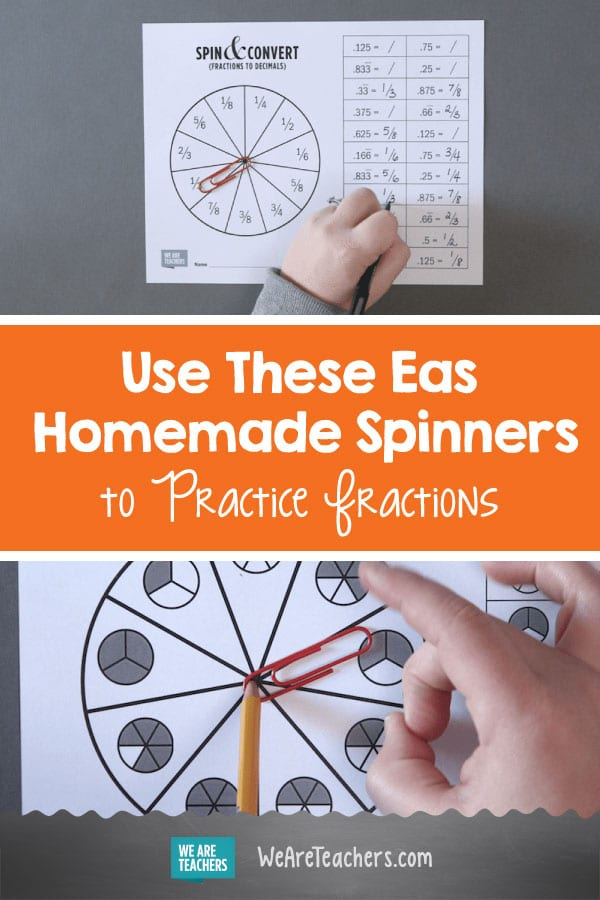## You Might Also Like